Chapter 9. Chemical Bonds

# Valence Bond Theory and Hybrid Orbitals

Jessie A. Key

### Learning Objectives

• Gain an understanding of valence bond theory.
• Gain an understanding of hybrid orbitals.

## Valence Bond Theory

Earlier we saw that covalent bonding requires the sharing of electrons between two atoms, so that each atom can complete its valence shell. But how does this sharing process occur? Remember that we can only estimate the likelihood of finding an electron in a certain area as a probability. This probability is represented as a distribution in space that we call an atomic orbital (Figure 9.5 “Representations of s and p atomic orbitals”)

Figure 9.5. Representations of s and p atomic orbitals.

The valence bond theory states that atoms in a covalent bond share electron density through the overlapping of their valence atomic orbitals. This creates an area of electron pair density between the two atoms. Since these electrons are simultaneously attracted to both nuclei, the electron pair holds the two atoms together.

Let’s examine the simplest case of atomic overlap resulting in a covalent bond, the formation of H2 from two hydrogen atoms (Figure 9.6 “A diagram showing the overlap of s orbitals of two hydrogen atoms to form H2“). The 1s orbitals of the two hydrogens approach each other and overlap to form a bond that has cylindrical symmetry known as a sigma bond (σ bond). Repulsion forces between the two nuclei and between the two electrons are also present. The optimal distance between atoms, which maximizes the attractive forces and minimizes the repulsive forces, gives the H-H sigma bond a length of 74 pm.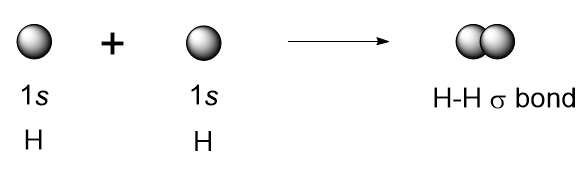Figure 9.6. A diagram showing the overlap of s orbitals of two hydrogen atoms to form H2.

For molecules that contain double or triple bonds, one of these bonds is a sigma bond, and the remaining multiple bonds are a different type of bond known as a pi bond (π bond). Pi bonds result from the sideways overlap of p orbitals, placing electron density on opposite sides of the internuclear axis (Figure 9.7 “Pi bond diagram showing sideways overlapping of p orbitals”).

Figure 9.7. Pi bond diagram showing sideways overlap of p orbitals.

## Hybrid Orbitals

### sp3 hybridization

A problem arises when we apply the valence bond theory method of orbital overlap to even simple molecules like methane (CH4) (Figure 9.8 “Methane”). Carbon (1s2 2s2 2p2) only has two unpaired valence electrons that are available to be shared through orbital overlap, yet CH4 has four C-H σ bonds!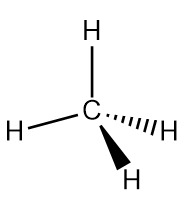Figure 9.8. Methane.

Figure 9.9. Nobel laureate Linus Pauling.

In 1931, Linus Pauling (Figure 9.9) proposed a mathematical mixing of atomic orbitals known as hybridization. The 2s and three 2p orbitals are averaged mathematically through hybridization to produce four degenerate sp3 hybrid orbitals (Figure 9.10 “Hybridization of carbon to generate sp3 orbitals”). Note that in hybridization, the number of atomic orbitals hybridized is equal to the number of hybrid orbitals generated.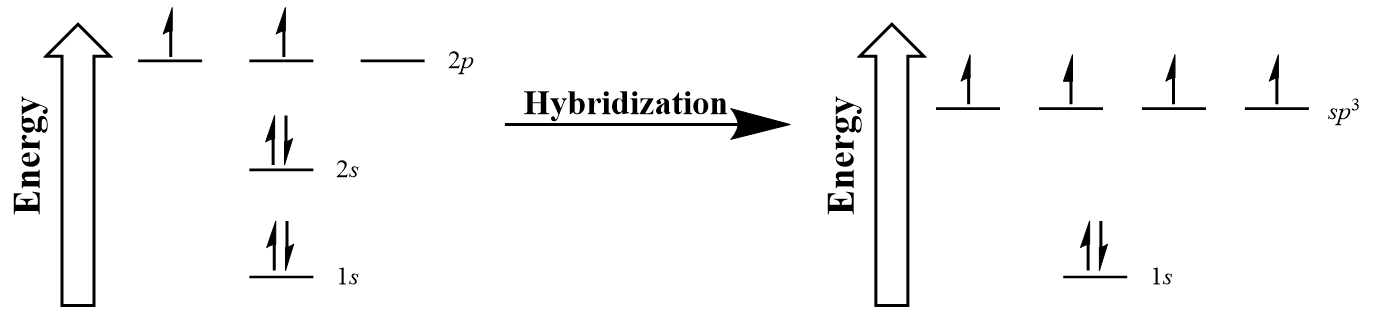Figure 9.10. Hybridization of carbon to generate sp3 orbitals.

The sp3 orbitals, being a combination of a spherical s orbital and propeller- (or peanut-) shaped p orbital, give an unsymmetrical propeller shape where one lobe of the orbital is larger (fatter) than the other (Figure 9.11 “An sp3 hybridized atomic orbital”). This larger lobe is typically used for orbital overlap in covalent bonding.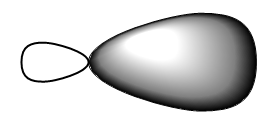Figure 9.11. An sp3 hybridized atomic orbital.

According to VSEPR theory, the four degenerate orbitals will arrange as far apart from each other as possible, giving a tetrahedral geometry with each orbital 109.5o apart (Figure 9.12 “A carbon atom’s four tetrahedral sp3 hybridized orbitals”).

Figure 9.12. A carbon atom’s four tetrahedral sp3 hybridized orbitals.

### sp2 hybridization

Let’s examine another simple molecule, ethene (C2H4) (Figure 9.13 “Ethene”). Each carbon of ethene is bonded to two hydrogens and a carbon. There is also a double bond between the two carbons.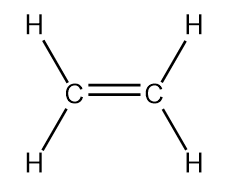Figure 9.13. Ethene.

Both the unhybridized atomic orbitals of carbon and the sp3 hybridization we just examined do not explain the bonding observed in ethene. In this case, only the 2s and two of the 2p orbitals hybridize to give three new sp2 hybridized orbitals capable of forming the three σ bonds of each carbon in ethene (Figure 9.14 “Hybridization of carbon to generate sp2 orbitals”).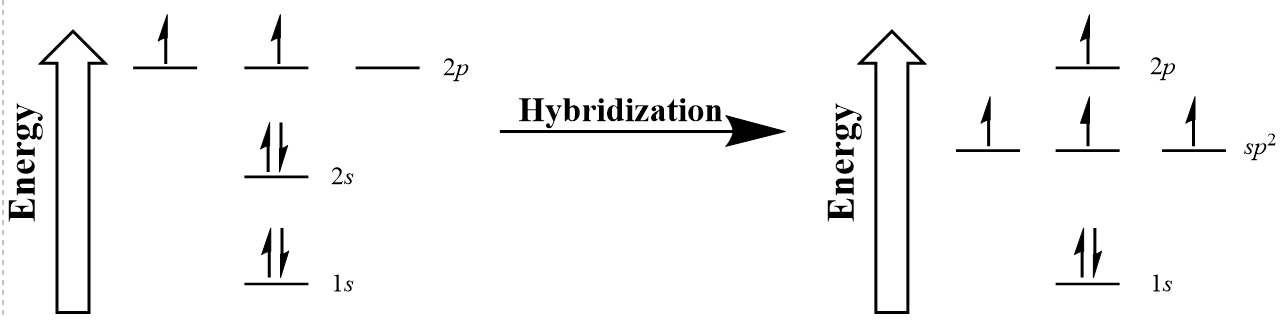Figure 9.14. Hybridization of carbon to generate sp2 orbitals.

The three hybridized orbitals arrange in a trigonal planar structure with a bond angle of 120o following VSEPR (Figure 9.15 “A carbon atom’s trigonal planar  sp2 hybridized orbitals”).

Figure 9.15. A carbon atom’s trigonal planar  sp2 hybridized orbitals.

The unhybridized 2p orbital in both carbons are left available to form the double bond’s π bond.

### sp hybridization

The final example of hybridization we will examine is the molecule ethyne (C2H2) (Figure 9.16 “Ethyne”).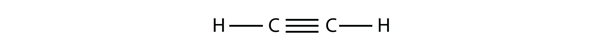Figure 9.16. Ethyne.

The carbons in ethyne are each sigma bonded to a single hydrogen, but triple bonded to each other. Again, the models of hybridization we have looked at so far are insufficient to explain the bonding pattern observed. In ethyne, only the 2s and one of the 2p orbitals hybridize to give two new sp hybridized orbitals capable of forming the two σ bonds of each carbon in ethyne (Figure 9.17 “Hybridization of carbon to generate sp orbitals”).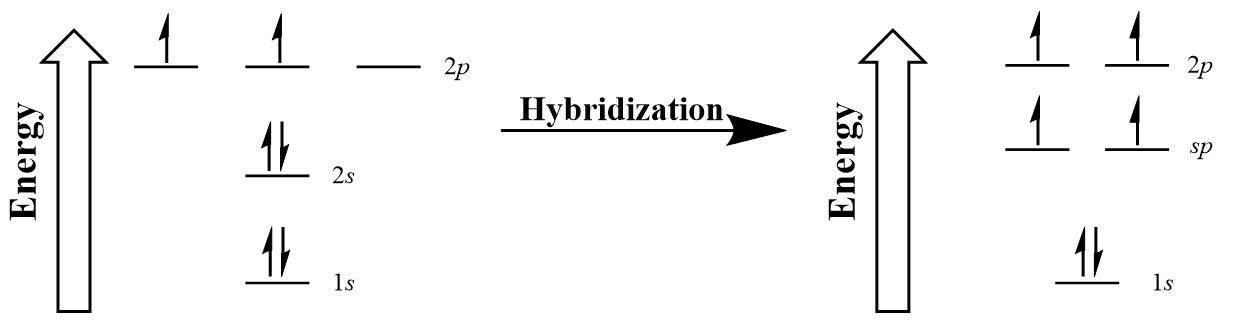Figure 9.17. Hybridization of carbon to generate sp orbitals.

The two hybridized sp orbitals arrange linearly with a bond angle of 180o following VSEPR (Figure 9.18 “A carbon atom’s linear sp hybridized orbitals”).

Figure 9.18. A carbon atom’s linear sp hybridized orbitals.

The two remaining unhybridized 2p orbitals in both carbons are left available to form the triple bond’s two π bonds.

### Other hybridizations

Other hybridizations are possible, allowing us to apply valence bond theory to explain the bonding patterns observed in most real molecules. Some additional hybridizations are summarized in Table 9.5 “Hybrid Orbitals and Geometry.”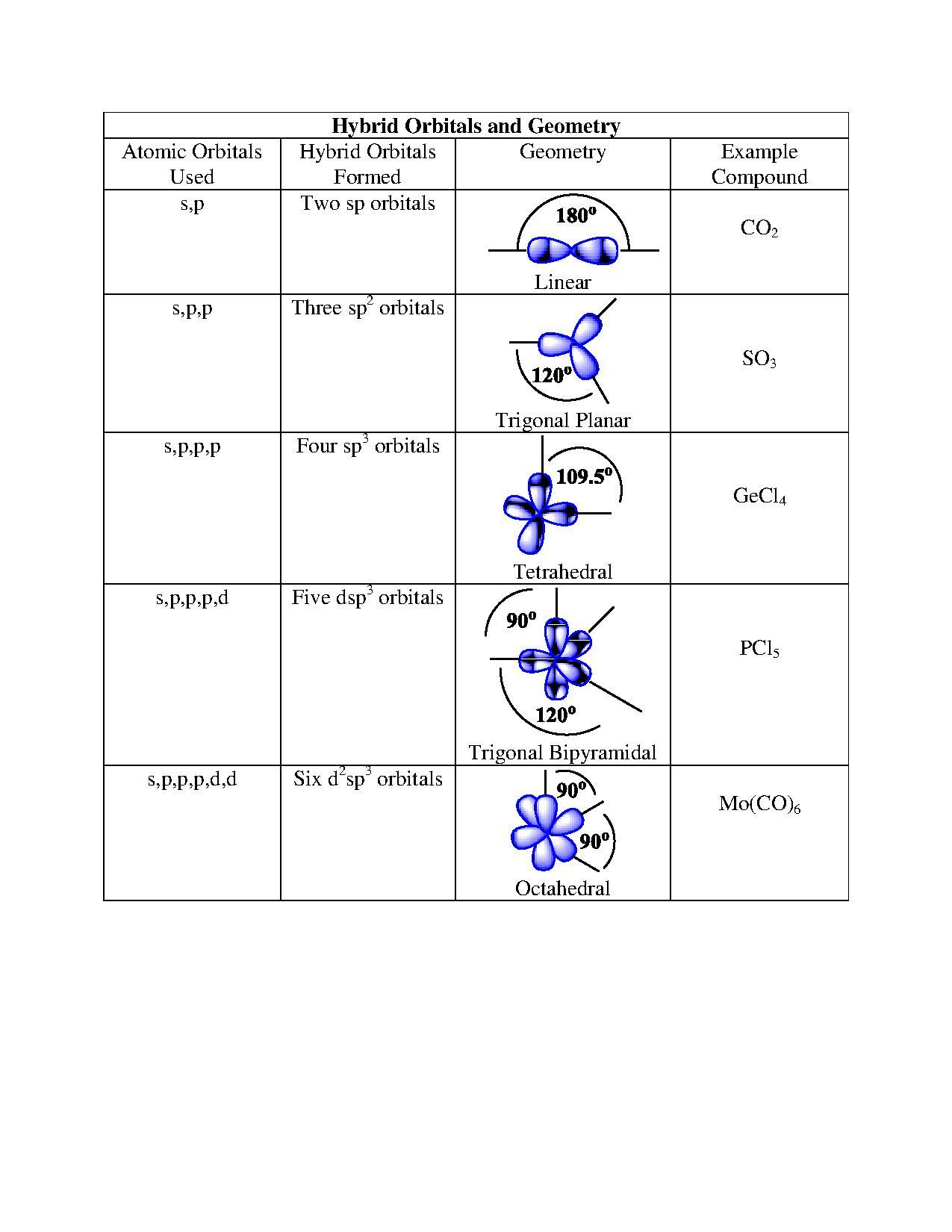Table 9.5. Hybrid orbitals and geometries.



### Key Takeaways

• Valence bond theory explains bonding through the overlap of atomic orbitals.
• Atomic orbitals can be hybridized mathematically to better explain actual bonding patterns.

1. This illustration shows all s and p atomic orbitals. By Sven\CC-BY-SA-3.0
2. Adapted from Pi-bond.jpg by JoJan\CC-BY-SA-3.0
3. L Pauling\Public Domain
4. Adapted from AE4h by Jfmelero\CC-BY-SA-3.0
5. Adapted from AE3h by Jfmelero\CC BY-SA 3.0
6. Adapted from AE2h by Jfmelero\CC BY-SA 3.0
7. Hybrid orbital and geometries by Chem507f10grp4\public domain.

## LicenseValence Bond Theory and Hybrid Orbitals by Jessie A. Key is licensed under a Creative Commons Attribution-NonCommercial-ShareAlike 4.0 International License, except where otherwise noted.